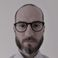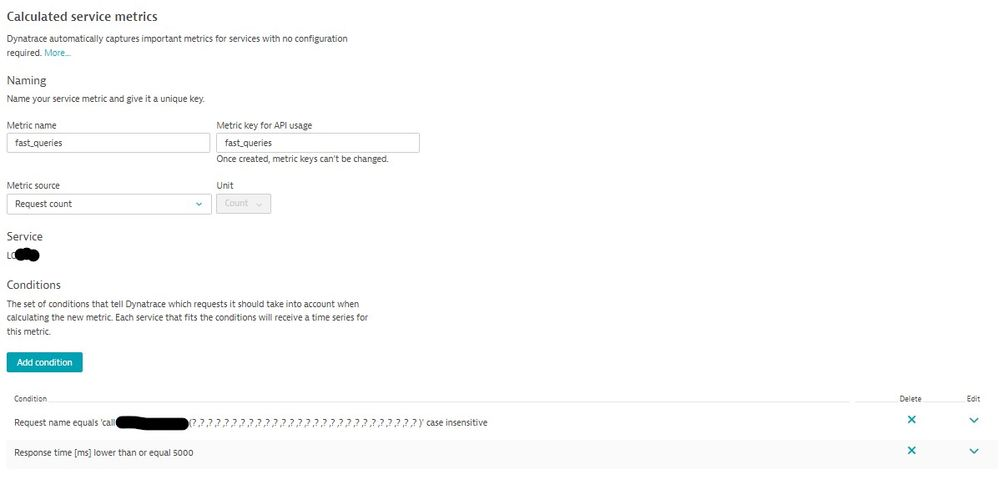cancel
Showing results for
Show  only  | Search instead for
Did you mean:Participant

I have a hard time finding the right documentation that describes how the aggregations and/or filters work on SLOs.
Here is my use case: get the ratio of calls under 5 seconds for a certain keyRequest.

((builtin:service.keyRequest.response.time:avg:partition("latency",value("good",lt(5000000))):splitBy():count:fold(sum))/(builtin:service.keyRequest.response.time:avg:splitBy():count:fold(sum))*100)

with a filter:  type(service_method),entityName("/apiY"),fromRelationships.isServiceMethodOf(type(service_method_group),fromRelationships.isGroupOf(type(service),entityId("SERVICE-Z")))

This setup is producing some numbers but are wrong. What am I missing?
Also, where can I find more info describing how the aggregations are suppose to work? Thank you

5 REPLIES 5Hi, that's very tricky and I'm not sure it can be achieved without implementing a calculated metric, see the example belowthe calculated metric will become your numerator, denominator will be the total request count for the mentioned service method.

Metrics API - Metric selector | Dynatrace Docs

ByeParticipant

I understand a computed metric (using DDUs) will provide the exact metric, but I want to understand what SLOs gives us.

It looks like the SLOs work on time slots (the finest time slot is 1 minute) and applies the desired aggregation at the time slot level.
The SLO definition require defining a timeframe, is that the time slot? or are the time slots defined as here: (https://www.dynatrace.com/support/help/dynatrace-api/environment-api/metric-v2/get-data-points#param...). I see the resolution depends on the query timeframe and the age of the data but if not specified it can use a default time slot of 120 data points.

If we consider the later, and assume we generate 120 data points per hour, and the default time slot is 120 data points, given the default SLO metric performance definition: ((builtin:service.response.time:avg:partition("latency",value("good",lt(10000))):splitBy():count:default(0))/(builtin:service.response.time:avg:splitBy():count)*(100)), will generate 2 values:
Value1 : corresponds the first 120 data points in the first hour = average(first 120 points)/120*100

Value2 : corresponds the next 120 data points in the second hour = average(last 120 points)/120*100

Will the SLO for two hours be the average of Value1 and Value2?

ThanksHi, not 100% to get your question but the SLO is evaluated in a moving timeframe. For instance if you define a 1 day evaluation (-1d in the settings) every SLO datapoint/value will be evaluated with respect to the previous 24 hours. Then, the metric created within the SLO can be combined as a normal metric (so if apply an avg aggregation on such metric on a larger timeframe you'll get the average during that timeframe).

Regarding the metric, one hint:

builtin:service.response.time:avg:partition("latency",value("good",lt(10000))):splitBy():count:default(0)

you are just defining the partition "good" but you're not filtering only "good" response time entries. You should add a :filter transformation to filter by latency equals to "good".

By the way I still suggest you to use a calculated metric for this.

ByeParticipant

Thanks for the feedback but not sure I am any clearer on how the "Service Performance"-SLOs works.
Let's use an example with a -2h timeframe and an app/setup that generates 200 monitoring data points (values) in two hours as follow:

• 120 values the first hour with 20 points < 10000 (with the average of all 120 values be 12000)
• 80 values the second hour with 40 points < 10000 (with the average of all 80 values be 5000)

How will the Service Performance SLO be computed?

Let's use this formula:
((builtin:service.response.time:avg:partition("latency",value("good",lt(10000))):splitBy():count:default(0))/(builtin:service.response.time:avg:splitBy():count)*(100))
Btw: the above was auto generated when selecting the "Service Performance SLO". My understanding is that if there is only one partition-value we don't need to have a filter for it. If am wrong, why doesn't Dynatrace add the filter to the autogenerated formula?
Anyway, even if we add the "good" filter, as you suggested, the main question is how does it work?

If we use method(1) - that has 120 data points per time slice - then a formula could be:

Value1: 20/120*100=16.6%
Value2: 40/80*100=50%
The SLO for the 2 hours could be: (16.6+50)/2 =33.3%

If we use a method(2) that takes into account the entire time window of 2 hours, the  SLO for 2 hours could be (20+40)/200=30%.

If all time slots have a fix count (and ignoring the edge time slots) the two methods produce the same value. But as I understood the time slots can be based on time – not clear when that is used.

Another method(3) could be that it uses the average of all the response times in the time slot. Then SLO in this case will 0 (by checking if 12000 is less than 10000) for the first time slot and 1 (by checking if 5000 is less than 10000) for the second time slot and the SLO  for 2 hours could be: (0+1)/2=50%

If none of the above 3 methods is accurate, using the above example can you please provide the expected SLO value (for 2 hour window) and the formula?

Lastly, if the same SLO definition (with 2 hour time window) is applied over 6 hours, is the expected value the average over the 3 time windows?

Thanks

Again, I understand that using a calculated metric will be more clear, but I would like to understand how the Service Performance SLO works and what it does.SLO works this way. Let's suppose that you want to check whether login time on your website lasts less than 10 seconds for at least 80% of your users.

SLO definiton will be: